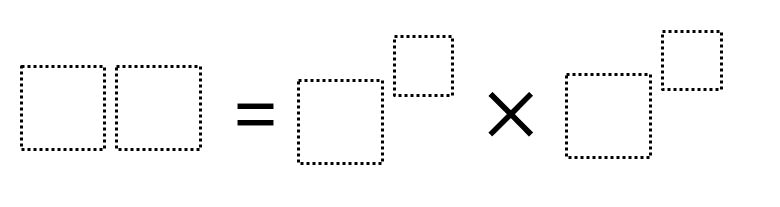# Prime Factorization 2

Directions: Using the digits 0 to 9 at most one time each, fill in the boxes to make the greatest possible product.### Hint

What digits would make good/bad exponents?
What digits would make good/bad bases?

The greatest product that’s been found so far is 80 = 5^1 * 2^4.

Source: Robert Kaplinsky

## Greatest Common Factor

Directions: Using the digits 0 to 9 at most one time each, place a digit …

1.2.we got 81= 9^2 x 7^0

•81 multiply by zero is zero

•But anything (except 0)to the power of 0 is 1, not 0.

•3.i did _____________=9^8×7^6

•80=5^1×2^4

•I 96 because 6^1 × 4^2 is equal to that.

•You can only use each digit once. You used the 6 twice.

4.i did 96=2^5+3^1

5.6.Why not 96=2^5+3^1

7.8.Danitza Marenco

That´s exactly what i thought 96=2^5+3^1

9.I think it would be 80 = 5^1*2^4

•5.0644e12 =

10.5.0644e12 =

11.96=2^5 x 3^1

12.80=5^1 * 2^4

13.14.You did a terrific job! This post seem extremely great.

15.I am not very sure but i am guessing that it would be 93= 2^5* 3^2 This is kinda confusing to me so i think i might be a bit off

•Maureen O'Donoghue

that gives 32 * 9 = 288 which is not a 2 digit number and uses 8 and 2 twice

16.I did 9^2*3*2=

17.I got 96 = 6^1 x 4^2

•You used the digit 6 twice.

18.I did 64=1^0*8^2 because anything to the power of one equals zero.

19.50 = 2^1 * 5^2
96 = 2^5 * 3^1

•Your first one doesn’t work, because you can only use each digit once, and you used 2 twice.

20.up i did 8⁹*6⁷ and got 37,572,373,905,408 i think i did something wrong oops

21.I love that one box represents only one digit. This is also a great exercise in order of operations. Thank you! It was also great that I realized the larger digits had to be the product AND the product had to be less than 100.
4^2 x 5^1 = 16 x 5 = 80
but even larger
2^5 x 3^1 = 32 x 3 = 96

22.I got 96=2^5*3^1 which is 32×3

23.2^5*3^1 = 96

24.we did addition in sted of multiplication so I got 4^3+2^5

25.I don’t think anyone has 40 = 2^3 x 5^1 which uses all of the numbers from 0-5!